# DIAGRAM display function

Contents of this document

1. Overview, introduction
2. Diagram control window - a dialog screen with three tabs:
1. Layout and preview
2. Channels, Mode, Timebase
3. Special diagram options (e.g. background image for the curve area, etc)
4. Configuration of a 'fast sampling' diagram using the Data Acquisition Unit (DAQ)
3. Zooming and scrolling via touchscreen
4. Commands to control a diagram during run-time (start,stop,clear, min,max)
5. Polygons in diagrams
6. Diagram-specific event handling in the application's script
7. Syntax for a diagram in a backslash sequence

How to...

## 1. Overview and introduction

The diagram functions are supported on any device with a graphic display, e.g. MKT-View III, IV, and many others. On devices with touchscreen, diagrams can optionally be controlled by the user (zoomed, panned) as described later.

On monochrome displays, diagrams looked like this: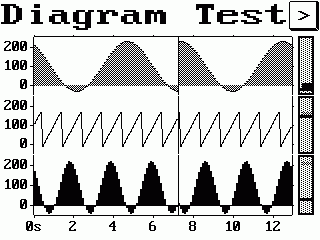Y(t) - diagram with 3 channels,
with 'windscreen wiper' instead of scrolling display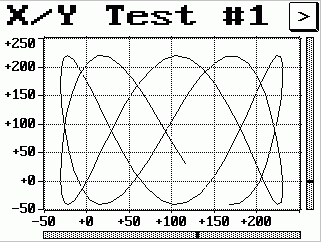X/Y - diagram with grid overlay

Other examples for Y(t)- and X/Y-diagrams can be found in the applications programs/diag_tst.CVT (for monochrome displays), programs/MKTview2/MV2_Demo.cvt (for colour displays with 480*272 pixels), and programs/MKTview4/MV4_Demo.cvt (for colour displays with 800*480 pixels) .

To insert a diagram on a display page in your application, either pick a page with an already existing diagram from the display page gallery, or create your own as described here:

• With the right mouse button, click into the LCD simulator screen (approximately at the position where the new diagram shall be inserted)
• In the popup menu which opens after this, select "Insert Y(t) Diagram"
• The programming tool will create a "default" diagram, which can be tailored on a special dialog screen ("Diagram properties", explained in the next chapter).

Alternatively, as for any other display element, diagrams can be inserted on a display page with the graphic editor, which may also be used to adjust the element's size or position (drag via mouse).

The dialog control window contains a preview of the diagram, as it would look like on the real terminal. You can also open this dialog by double-clicking on an already existing diagram in the LCD simulator window. The diagram control window will be explained in the following chapter.

## 2. Diagram control window (dialog)

To modify specific properties of a diagram, you can open the following dialog window. It is divided into several tab sheets, where the diagram's layout, channels, and some special options can be modified.

Note: In some terminals it is possible to draw polygons as an overlay over the diagram area. The polygons must be drawn with an interpreter- or script command, they cannot be added to the diagram during desing time (in the diagram control window).

### 2.1 Layout and preview

The first tab sheet of the diagram control dialog is used to define the general layout, and it contains a preview showing what the diagram would "look like" with the current settings: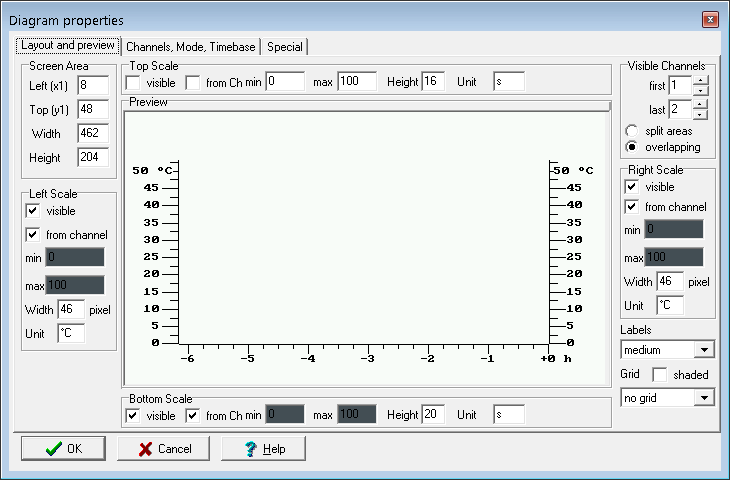The Screen Area defines position (x1,y1) and size (width,height) of the diagram area. Changes in these fields immediately affects the preview.

The four scale controls on this tab define if and how axises are drawn at the left, right, top or bottom edge of the diagram area. The "size" parameter is either the width or height of the scale area, measured in pixels.
If a checkmark "from channel" is set, the value range for the scale labels is taken from the channel definition (on another tab). If it is not set, you can enter arbitrary values which do not necessarily match the value range of the parameter. This is frequently used to draw a "percentage" scale, for example if multiple channels with different scaling (or units) shall be displayed in the same diagram.
If the option "from channel" is not checked, you cannot enter arbitrary min/max values a scale because those parameters will be copied from the channel definition; and for the horizontal scale at the bottom of an Y(t) diagram, the default unit will be "s" (seconds).

Note:
Changing the style of the scale-indicator does not affect the displayed value range ("zoom factor") of the graph ! To change the displayed value range, use the "min"/"max" values on the tabsheet "Channels".
A physical unit can be entered with a maximum length of 7 characters (in the field labelled 'Unit' / 'Einheit'). This unit will only be displayed in one label of a scale.
The unit 's' (for 'seconds') in an Y(t)-diagram's horizontal scale means this scale shall display the time, relative to the current time (which would be zero on this time scale). Depending on sampling rate and horizontal zoom, the firmware may decide to use hours (h) or minutes (min) instead of seconds when drawing the time scale.
For other kinds of scales (without unit 's'), the firmware may automatically add one of the following prefixes (before the unit), depending on the visual scale range (min, max):
n
nano ( X * 10^-9 )
u
micro ( X * 10^-6 )
m
milli ( X * 10^-3 )
k
kilo ( X * 10^3 )
M
Mega ( X * 10^-6 )
G
Giga ( X * 10^-9 )

On tab 'Visible Channels' you select which of the 8 diagram channels shall be visible in the current diagram (there is a total of 8 channels; shared by all diagrams of your application as explained later). In X/Y-mode, the first channel will be the "X"-channel, the second the "Y"-channel. In Y(t)-mode, the first channel will be the upper curve if 'split areas' are selected. In 'overlapping areas' mode, all curves are plotted into a common diagram area - which can be confusing on a monochrome display sometimes. You should use different channel graph styles in this case as explained later.

Three different font sizes can be selected to add Labels to the scales of your diagram, or -alternatively- the numeric labels can be turned off (for all scales of the diagram) by selecting "no labels" in the "Labels" combo.
Note: If the 'size' of a scale is very small, the labels are automatically turned off for that particular scale. The drawing routine will not try to squeeze numeric labels into a too small scale area. This trick was used in the example shown above, where the sizes of the upper and right scale are only two pixels.

An optional grid can be turned on for the diagram's graph area. You can select no grid, vertical, horizontal, or both. The grid can be drawn either as solid lines or "shaded" (which is a fine-pitched dotted line for monochrome displays, which appears gray from some distance).

### 2.2 Channels, Mode, Timebase

On the second tab sheet, the data channels and some other diagram options can be defined: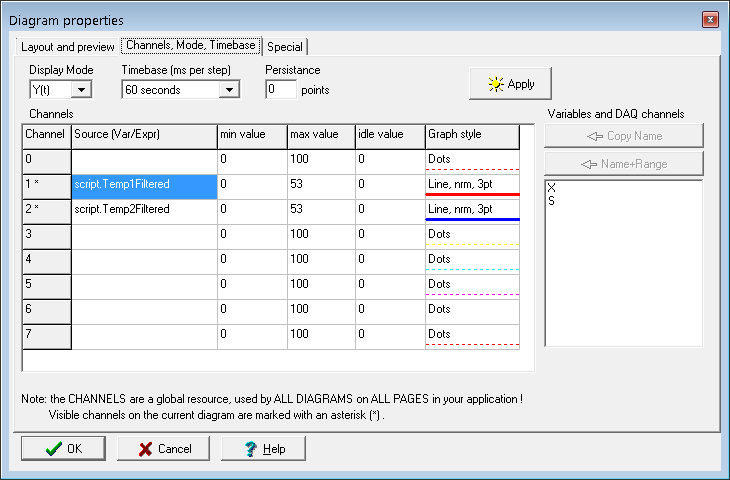The controls on this tab sheet are:

Display mode
Can be set to Y(t) or X/Y. In X/Y-mode, the first channel of the diagram controls the X-axis, the second channel the Y-axis. In Y(t)-mode, the time dependent behaviour of all channels of the current diagram is displayed (X-axis = time).

Timebase
defines the update interval (usually a few ten milliseconds). In Y(t)-mode, a horizontal step of one graphic pixel is done per interval. In X/Y-mode, this interval - together with the "Persistance" value- defines how long old it takes until old points disappear from the screen (unless the special option "don't clear old curve" is selected).
If any of the diagram's channels is connected to the DAQ-unit, then the DAQ-unit's sampling rate may be automatically adapted to match the diagram's timebase.
Don't miss the notes about 'fast sampling' diagrams here !

Persistance
Only used for X/Y mode. Defines how many "old points" (or polygon segments) are visible on the screen. Must be between 1(*) and 255. If, for example, the Timebase parameter is set to 5*10 milliseconds, and "Persistance"=100, a new point will be drawn every 0.05sec, and will disappear from the diagram screen after 5 seconds (100 points * 0.05 seconds).
(*) Note: A persistance value of 1 (ONE) is only allowed if the graph style is set to "single dots". If the points of the graph shall be joined with lines, the persistance value must be 2 (TWO) or more.

Source (in the diagram-channel definition table)
Defines the origin of the samples in this channel. Usually, this will be the name of a display variable, but it's also possible to enter a short numeric expression in this column (which may call one of the interpreter's numeric functions).
By virtue of the DAQ unit, diagrams can also plot the waveform from an analog input (onboard), with a relatively high sampling rate (as far as the A/D converter + the DAQ permit).
Besides display variables and DAQ channels, diagrams can also directly show values from arrays declared in the script. References to such arrays are listed as script.<name>, where <name> is the name of a script variable declared as a one-dimensional array.
Note about plotting data from script arrays.

Min Value, Max Value (in the diagram-channel definition table)
Defines the graphic display range for a channel. You can use this to zoom into a certain range of interest. This range may affect the numeric labels on a vertical scale (if the checkmark "from channel" is set for a vertical axis).
When defining a new data channel, the min/max value are usually copied from the propierties of an UPT-variable, because a diagram input channel is usually connected to one of the UPT's user-defined variable. If you want to display more than one channel in the same diagram area, you should use a common value range. If this is not possible, use a simple common scale ("percentage") for the diagram and clear the checkmark "from channel" on the control panel for left+right scale.
For very special applications, you can also set (overwrite) these parameters with an interpreter command.

Graph Style (in the diagram-channel definition table)
Defines how the samples from this channel shall be drawn on screen (line style and thickness). In Y(t)-mode, this may be "single dots", "lines" (vectors, drawn-through or dotted), "black fill" or "gray filling pattern". To modify this, double-click into this cell (row+column) of the definition table. In X/Y-mode, only "dots" and "lines" are supported (but no filled areas). The line strength ('thickness') also applies to single dots. For example, this allows you to have a single, large dot moving around the screen (use persistance=1 for this).

Copy Name / Copy Name+Value Range
Copies the name of a variable (and optionally its value range) from the list of variable names into the currently selected channel definition line.

Notes:

• The "source" for a diagram data channel is usually a variable, but that is not necessarily the case. You can enter any numeric expression in the "Source" column, for example "sys.vsup" if you want to plot the terminal's own supply voltage, etc etc.
• The values shown in a diagram are internally stored in an array ('sample memory'). Data acquisition into these arrays starts as soon as a diagram gets visible. Data acquisition into these arrays begins shortly after power-on (the firmware scans all display pages for diagram channel definitions, which contain info about the "source" and the desired sampling rate). If the source is a normal variable, the array will be updated (in the background) even if the page with the diagram is not visible. As soon as the the page with the diagram gets visible, the diagram will be updated using the sampled values stored in the channel's internal array.
• Without the aid of the Data Acquisition Unit (DAQ), the maximum sampling rate is limited by the speed of the main loop. Depending on the complexity of the display, a main loop may take 100 milliseconds. Thus to achieve sampling rates of 10 Hz (and more) without drop-outs, use the DAQ as source (sample memory) as explained in chapter 2.4, for all channels that require 'fast sampling'.
• If the plot data are available as an array (in the script), the array-variable can be connected directly to a diagram channel as shown further below. For example, see application 'programs/DAQ_Test.cvt'. In that application, the script calculates the spectrum of an input signal (using the FFT), logarithmizes the amplitudes (converts into Decibel), and lets a diagram plot the contents of the array whenever a new calculation is finished. To use the array (hier: fltLogSpectrum with the logarithmized amplitude spectrum) as 'source' for one of the diagram channels, use the selection list shown below.Selection list with data sources for a diagram, including a few script variables (arrays) To 'connect' a diagram channel to a script-array at design time, first select the name in the list (see screenshot above), then click button 'Copy Name'. The designer doesn't know the range of values in the array (because it's your script filling such an array at runtime, not at design-time), thus the button 'Copy Name + Range' is disabled if the currently selected list item is an array in your script. So enter the min/max value range manually in the designer, unless you set it later at run-time via script (display.dia.ch[].min, .max).

All sources with the prefix 'script.' are collected from the script after compilation. Only suitable arrays will be listed, for example 'fltFFTin', 'fltFFTout', and 'fltLogSpectrum' from the following code snippet (taken from DAQ_Test.cvt):
```const C_FFT_SIZE=1024; // number of samples for the FFT in the time domain, 2^N endconst; ... var float fltFFTin[C_FFT_SIZE]; // FFT input (N real time domain samples) float fltFFTout[C_FFT_SIZE+2]; // FFT output (N+1 complex frequency bins for the REAL FFT) float fltLogSpectrum[C_FFT_SIZE/2]; // logarithmized amplitude spectrum (dB "over" full scale) float re,im,mag,ref; int i; endvar; ... daq.read_channel( 7, fltFFTin ); // read input for the FFT from the DAQ into an array Math.rfft( fltFFTin, fltFFTout ); // Fourier Transform with real input, complex output ... display.UpdateDiagram; // update diagram AFTER the new set of data is complete ... ``` To force an update of the diagram after calculating new values (in an array declared in your script), use the command display.UpdateDiagram. When plotting Y(t)-diagrams with array data as in the example shown above, the diagram doesn't "know" the sampling rate. Furthermore, in this case, the diagram also doesn't know that the horizontal axis should be labelled in Hertz (for the spectrum display) rather than seconds. Thus, without further actions in the script the diagram will plot one array element per pixel (horizontally), and the horizontal axis appears like a 'time axis' (unit: seconds or milliseconds), even if the curve shows samples in the frequency domain, not in the time domain.
To change the labels shown in the horizontal axis to "Hertz" (or Hz, or kHz), open the tabsheet Layout and Preview, and uncheck option 'from Channel' for the 'Bottom Scale'. Now you can manually modify the value range and physical unit for the labels, instead of letting the diagram select range and labels automatically.

### 2.3 Special diagram options

On the third tab sheet, some 'special' options can be selected.(Screenshot of the 'Diagram' dialog with the 'Special' tab opened)

Up to now, the following special options can be modified on the 3rd tab:

• don't clear old curve
Can be used for unattended long-term observations. It may help you to see sporadic "runaways" (like unusual peaks in an otherwise flat line).
• draw "wiper" at current position ("windscreen wiper")
Draws a thin vertical line at the current plotting position in Y(t)-mode. No effect in X/Y mode.
Since 2016-08-04, the wiper's horizontal position is not reset when re-drawing a display page completely anymore. Instead, the position is only reset when switching from one display page to another, or when clearing the curve via command dia.clear.
If the diagram is configured as 'scrolling', the windscreen wiper has no function, because new data always appear at the right edge of the diagram.
Note about 'Windscreen Wiper' vs 'scrolling display':
The non-scrolling 'windscreen wiper' is an anachronism for old 'passive STN' LCDs, and only still supported to remain compatible with old devices like 'MKT-View I'. The TFT displays in modern devices are fast enough even below the freezing point, thus for new applications, don't use the 'non-scrolling display with windscreen wiper' anymore. Use the scrolling display instead (details further below).
• static display (no periodic update)
For 'very special' applications, in which the display shall not be updated (redrawn) 'automatically', but (for example) only via command in the script.
• scrolling display (only for Y(t) diagramm)
With this option, the entire curve plotting area scrolls along the timescale (from right to left). The latest (newest) sample will always appear on the right edge of the plotting area.
No effect in X/Y mode.
• scroll- and zoomable via touchscreen
Allows the operator to modify the displayed value range at runtime. For details, see chapter 3.
• start acquisition only if this diagram gets VISIBLE
Check this option if you need the old behaviour of a diagram, where data acquisition (for all channels connected to the diagram) did not start immediately after power-on.
• enable debugging
Please don't. This option is only intended for software development.
• Background image (tCanvas)
Optional image to fill the background of the diagram's curve area, generated by the script at runtime. Without a background image, the curve area will be filled with the background colour.
In the following screenshot from application DAQ_Test.cvt, the diagram shows the microphone input in the time domain (red trace), the momentary spectrum (green trace), and the chronological sequence of audio spectra as a spectrogram (aka 'waterfall display') in the background of the curve area. For that purpose, the script uses a tCanvas for the spectrogram and the fast Fourier transform (FFT) to calculate the short-time spectra.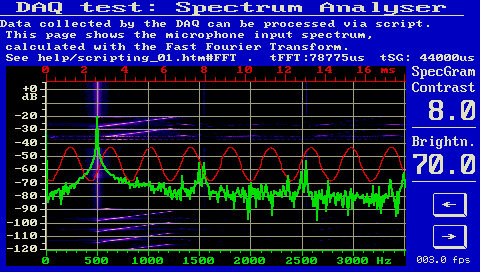Example for a diagram with background image (here: spectrogram from 'DAQ_Test.cvt')
More examples with the options listed above are in the application "diag_tst.cvt" .

### 2.4 Configuration of a 'fast sampling' diagram using the Data Acquisition Unit (DAQ)

As already mentioned in chapter 2, channels with sampling rates above 10 Hz (i.e. sampling intervals below 100 milliseconds) should be "collected" (sampled) via DAQ unit. The DAQ uses interrupts instead of polling, and can achieve sampling rates as specified in the DAQ documentation. The DAQ can be configured on the programming tool's 'Variables' tab as explained here, but since the DAQ also supports other signal sources (that are not 'variables'), the DAQ can be fully configured through the programming tool's main menu under 'Options'..'Data Acquisition Unit'.
Alternatively (if an already existing diagram shall be reconfigured to use the DAQ), you can use the button labelled     'Connect XYZ via DAQ'     (with 'XYZ'=name of the source)
on tabsheet 'Channels, Mode, Timebase' as explained here.

## 3. Zooming and panning (scrolling) a diagram via touchscreen

In devices with touchscreen, the operator may zoom into the diagram, or pan (scroll) the displayed value range as explained further below. To offer this feature, on the 'Diagram Properties' dialog, switch to the 'Special' tab, and set option
scroll- and zoomable via touchscreen.

When enabled, the option allows to control Y(t)-diagrams as follows:

• Via touchscreen, vertical scales can be grabbed and moved up and down to shift the displayed display range of the ordinate ("Y axis").
The diagram's curve are will be scrolled along with the scale(s).
• In Y(t)-diagrams with a visible horizontal time scale (i.e. physical unit set to "s" for "seconds"), the diagram can be shifted along the time axis by grabbing and moving the time scale via touchscreen. If data for the shifted range are available in the channel memories, the curves will scroll along with the timescale. Otherwise ('no data available' for a certain point in time), the curve remains empty, but will eventually be filled since the diagram's data acquisition keeps running in the background.
As explained in chapter 2, a time scale with unit set to "s" in the designer doesn't mean the labels will always show 'seconds' - instead, the labels may show milliseconds, minutes, or even hours instead. But in any case, the value 'zero' represents the current date and time ("now") on this 'relative' timescale, and negative values represent the past.
• To zoom in and out, press the touch pen (or your finger) on the scale two times in a short time (*). On the second tap, hold it pressed. Then move the pen up to zoom in (expand), or down to zoom out the displayed range.
The initially touched position remains in the center of the zoomed range.
• (with multi-touch capable hardware, you would be able to zoom into the diagram as you would on a smartphone or tablet PC, by two-finger-spreading gesture over the to-be-magnified object. But at the time of this writing (2018-08), only a single prototype with multi-touch display existed.)
• To switch back to the original ("designed") display ranges, rapidly (*) tap on the diagram's scale three times.

* To recognize double or triple clicks from the touchscreen, the time
between two pen-down events must not exceed 500 milliseconds.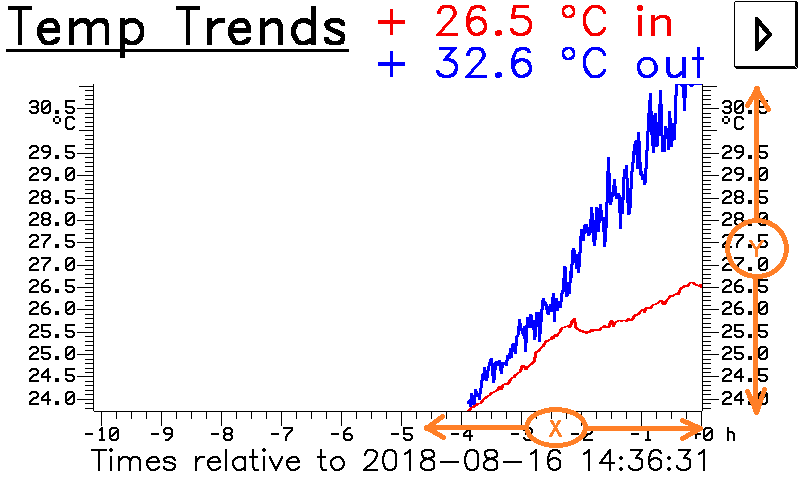Scrollable Y(t) diagram from application script_demos/Thermo.cvt.
Orange circles and arrows mark the touchable areas for zooming,
or for scrolling along the horizontal (X) or vertical axis (Y).
Touchscreen events inside the curve area are reserved for the 'readout' feature (marker, cursor).

### 3.1 Sample memory (for zooming and scrolling diagrams)

At the time of this writing (2018-08-17), the memory for each diagram channel was limited to 2048 samples. If more memory is required (e.g. to 'scroll back in time' by more than 2048 sample points), you could use a sufficiently large array (declared in your script) to provide more data for scrolling back.
If there is sufficient demand from users, even a callback function (from diagram to script) can be implemented to supply data for plotting (read from file, etc).

## 4. Commands to control a diagram at runtime

The following interpreter-commands can be used to control the diagram display during run-time.
To invoke them from the script language, the commands must be prefixed by 'display.' .
At the moment, these commands are only available for the display interpreter, but not in the script language.

`dia.clear`             (display interpreter command)
`display.dia.clear`      (script command)
This command erases the old graph in the diagram. In Y(t)-mode a new scan begins at the left side of the graph area. The grid (if enabled) will be redrawn immediately.

`dia.run=X`             (display interpreter command)
`display.dia.run := X`    (script command)
This command stops and restarts the diagram display routine. "dia.run=0" means "Pause" (no more update of the visible diagram), "dia.run=1" or simply "dia.run" means "end of the pause, continue to update the diagram".
The current state (diagram stopped / running) can be queried with the function "dia.run". The following example shows how to toggle the "running" state:
`dia.run=!dia.run`
(the operator "!" means "boolean negation").

`dia.ready`             (display interpreter function)
`display.dia.ready`       (script function)
This function returns the diagram's scan-complete flag. It can be used to implement a single-shot diagram which can be started by the user and stops automatically when the scan reaches the end of the timebase. Use `dia.ready` in an event definition, and `dia.run=0` as the reaction. This stops the diagram after a single scan. An example can be found in the file "diag_test1.cvt".
This flag is cleared automatically with the command `dia.clear` and `dia.run`.

`display.dia.unix_time`(script function)
Returns the absolute timestamp (in "seconds elapsed since 1970-01-01 00:00:00") of the sample displayed on the left edge of the curve area). Unless the Y(t)-diagram was 'scrolled back in time', the result is almost identical to
` system.unix_time - display.dia.timespan_sec`
Note: At the time of this writing, display.dia.unix_time was a read-only parameter. It cannot be used to horizontally scroll the diagram, but is affected by scrolling or zooming.

`display.dia.timespan_sec`(script function)
For Y(t) diagrams, returns the "visible width of the curve area" in seconds.
This read-only parameter depends on the width of the diagram in pixels, but also from the timebase setting, and the magnification via touchscreen.

`dia.plot_daq`          (display interpreter command)
`display.dia.plot_daq`    (script command)
Plots the most recent data collected by the data acquisition unit (DAQ) into the diagram.
Side effect: The diagram is switched into a 'static' mode, without dynamic plotting.

`dia.ch[N].min, dia.ch[N].max`                (display interpreter read- and writeable parameters)
`display.dia.ch[N].min, display.dia.ch[N].max`   (script read- and writeable parameters)
Access to the channel scaling parameters during runtime. Can be used to "zoom" into the diagram area. Overwrites the channel's min/max parameters which were designed at design-time. The channel index (N) is the same as on the "Channels, Mode, Options" tab, the index begins at 0 (zero) for the first channel !
To zoom into an X/Y diagram, you must assign new values to four of these parameters ! Depending on which channel is connected to the X- and which to the Y-axis, these may be `dia.ch.min,` `dia.ch.max`, ` dia.ch.max`, and `dia.ch.max` . The diagram will be automatically redrawn if you assign new values to the channel's scaling parameters.
To revert to the channel scaling parameters at design time, use the command `dia.ch[N].reset` .
When zooming or scrolling the diagram via touchscreen, these values may change at runtime.

`dia.ch[N].reset`                          (display interpreter command)
`display.dia.ch[N].reset`                    (script command)
Reverts to the channel scaling parameters at design-time. Can be used to "zoom out" after modifying the diagram's scaling parameters with the commands `dia.ch[N].min` and `dia.ch[N].max` .

`dia.sc.X.min,  dia.sc.X.max`                 (display interpreter read- and writeable parameters)
`display.dia.sc.X.min,  display.dia.sc.X.max`    (script read- and writeable parameters)
Modifies one of the numeric scales of a diagram during runtime, but not the transformation parameters for a data channel. X: l=left, r=right, t=top, b=bottom . Only works if the scale is not associated to a channel (which means, the checkmark "from channel" must not be set for this scale) ! Example:
`dia.sc.t.min = -1.5 : dia.sc.t.max = 100.0`

## 5. Polygons in the curve area of a diagram

Only available in certain terminals (see Feature Matrix): Display interpreter commands to draw polgons inside the graph area, using the same coordinate transformations like the graph ("curves"). Similar functions also exist in the script language, but the syntax is slightly different (and more versatile).
```dia.poly(from_row, to_row, from_column, to_column)```         (display interpreter command)
`dia.poly(arr[from_row~to_row][from_column~to_column])`   (display interpreter command)
`display.dia.poly[N].draw( <source_array>, <options> )`   (script command)
Draws a closed polygon in the plotting area of the diagram. The polygon coordinates are usually copied from an array (for the display interpreter, there's only a global array). The second notation (with the explicit specification of the array's name) is the preferred syntax (and in the script, the only possible form). Example for the display interpreter:
`dia.poly(arr[0~8][0~3])`
Draws nine polygons, using rows 0..8 in the global array; with four points each, stored in in columns 0..3 of the array.
Note: The array must be filled with the point coordinates before this command is invoked ! How to fill the array is explained in a separate document for the display interpreter, and here for the script language.
For individual control, you can specify which of the diagram's polygons(*) shall be drawn, and for clarity, you can add the token ".draw" after the polygon index. Example for the display interpreter:
`dia.poly.draw(arr[0~3],o)` draws polygon #9 only, using the 11-th line of the array, as an OPEN polygon (lower case 'o' at the end)

(*) The number of polygons per diagram is limited to 20, even though the capacity of the array which holds the polgon coordinates may be larger. Thus, the maximum polygon index -also for the commands further below- is 19 because array indices begin at zero.

`dia.poly.clear`                     (display interpreter command)
Removes all polygons from the diagram's plotting area.
`dia.poly[N].dmode=`<draw mode>         (display interpreter command)
`dia.poly.dmode`                     (display interpreter function)
Changes the display mode for the polgon on a monochrome display. N is the polygon index (counting starts at zero, N=0 is the FIRST polygon, max index is specified here). Usable draw modes are: 0=solid, not blinking, 1=blinking in page 1, etc). The polygon index can be left out to change the appearance of all polygons with a single command.
For colour displays, the 'draw mode' has no effect. Use different colours instead.
`dia.poly[N].color=`<color>             (display interpreter command)
Changes the the colour of the specified polygon's lines (if the device has a colour display).  If no particular polygon index (N) is specified, the command sets the colour for all polygons currently visible. An overview of all usable colours can be found here .

`dia.poly[N].width=`<line width in pixels>   (display interpreter command)
`dia.poly.width`
Changes the width ("thickness") of the polygon lines in pixel. The polygon index can be left out to change the appearance of all polygons with a single command. Use this feature economically, because too wide lines may slow down the screen update rate. The default line width is 1 (one) pixel.
`dia.poly[N].text=`"<string>"           (display interpreter command)
Prints a short text message (max. 8 characters) close to the specified polygon. The text must be specified with this command before the polygon is drawn into the diagram. Defining the string during runtime is not possible. Note that the number of polygons per diagram is limited.

Other commands to control the diagram may follow in future.
An example for advanced users with runtime-generated polygons in a diagram can be found in the application MKTview2/MV2_Poly.cvt.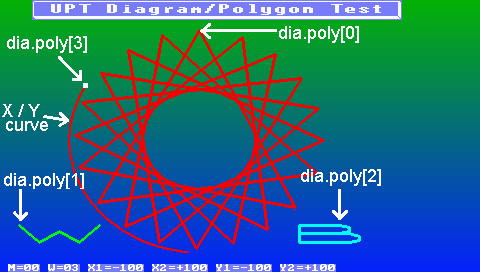Screenshot from application 'programs/MKTview2/MV2_Poly.cvt' (Test for diagrams with polygons in the curve area, here on an MKT-View III) The white dot in the screenshot above marks the most recent measurement (here: X, Y). It was realized as a 'polygon' consisting of a single 5-pixel large dot with the following display interpreter commands (on the display page under 'local events'):
```  Event     Reaction
always    arr[0~1]={X,Y};         // copy X and Y into the array (one point)
always    dia.poly.color = white : dia.poly.width = 5; // prepare drawing ..
always    dia.poly.draw(arr[0~1],o); // draw X,Y as polygon with a single point
```
In the sample shown above, the diagram itself is transparent, and the background is a colour gradient covering the entire screen. To remove old pixels (and moving polygons) from the screen, the display page is always redrawn completely (defined on the display page header).

### 5.1 Drawing polygons in diagrams via script

In October 2018, the old display interpreter commands for drawing polygons were replaced by a script command with the same purpose. The old interpreter commands only still exist (in the firmware) to remain compatible with existing applications.
New syntax (in scripts, since October 2018):
`````` display.dia.poly[Index].draw( <Source> [,Colour][,Text][,Options] )
|             |         |      |      |
|             |         |      |      |__ optionale flags
polygon index,       |         |      |
optional, 0..19      |         |      |__ optional text string, to be
|         |          displayed 'near the polygon'
|         |__ optional specification of a colour
e.g. an array, optionally with the number of points
``````
If (as used in the example presented further below) the source is an array, the next argument may be an integer value with the number of points to be plotted. Otherwise, the number of points is equal to the array's 'momentarily occupied length' (array.len).
More optional parameters may follow, like colour (a value with data type tColor), and a text string displayed near the polygon (a value with data type string).

Internally, polygon coordinates are still stored in the display interpreter's global numeric array ("arr"), and read from there whenever the diagram needs to be updated on the display. This may change in future.
The script language supports user-defined arrays, which are typically used to store the coordinates for the polygon points (these vertices use 'physical' scaled values, not pixel coordinates). A three-dimensional array can store coordinates for multiple polygons, while a two-dimensional array can only store coordinates for a single polygon (with N points).
The least significant array dimension (rightmost pair of squared brackets for the index) will be used as follows:
`Index 0` stores the horizontal coordinate (or, for Y(t)-diagrams, a timestamp in seconds),
`Index 1` stores the vertical coordinate ("Y"),
`Index 2` is reserved to store an additional "Z" component for future applications,
`Index 3` could be used to store individual 'flags' for each point (vertex).

So, for simple applications at least a two-dimensional array is required, with at least two components (one for 'X' and one for 'Y') in the least significant dimension. Example (declaration of a 3D-array for polygon coordinates in script_demos/diagrams.cvt):
``````
var
float PeakIndicator;
//            |  |  |___ coordinate : 0=x, 1=y (least significant dimension)
//            |  |______ point index (here, up to 5 points per polygon)
//            |_________ polygon index : 0..2 (for up to 3 polygons)
endvar;
``````
After filling the array with data (not shown here), it can be used to draw a polygon into the diagram as in this example from script_demos/diagrams.cvt:
``````
display.dia.poly.draw( PeakIndicator, 3, clRed, "Indicator 1", pfClosed );
//                         | source, here with|   |         |           |
//                         |  number of points|   Colour    Text        |
//                         |_______(optional)_|  (optional) (optional)  |
//  ____________________________________________________________________|
// |
// |
// Option
``````
The 'Option' may be specified as the last argument. It's an integer value, actually a bitwise combination of the following flags:
`   pfOpen` : Draw an open polygon
`   pfClosed` : Draw a closed polygon
`   pfNoScroll` : Draw a polygon that, for Y(t)-diagrams, does not scroll along with the curve(s).
Without this flag, for Y(t)-diagrams, the 'X' coordinates are absolute timestamps,
and thus they scroll along with the measured values from right to left.
Otherwise (with pfNoScroll), the 'X'-coordinates for Y(t)-diagrams
must be between zero (= left edge of the curve area) and N seconds (= right edge),
where 'N' (a value in seconds) depends on the width and timebase configuration.
This "visible width in seconds" (N) can be queried via display.dia.timespan_sec.

Examples for drawing polygons into diagrams, with detailed comments, can be found in the sample/test application
script_demos/diagrams.cvt.

Polygons outside a diagram, created with the graphic editor

## 6. Diagram-specific event handling in the application's script

For special applications with diagrams, a script may contain specialized event handlers(*), that are invoked whenever 'something special' happens with the diagram - most noteably, touchscreen events in certain areas of the diagram.
The names of these optional event handlers are fixed (hard-coded in the system), so if you need these handlers, implement them in your script exactly as shown in the examples below. If such a handler exists, it will be called if the option 'invoke event-handlers in the script' is set when designing the diagram on the 'special options' tab. This way, diagrams on certain pages can be excluded from this non-standard event-processing.

`func OnDiagramTouchEvent( `int event, int area, float scaled_x, float scaled_y `) // touch event in a diagram`

This handler will be invoked by any kind of touchscreen-event within the diagram's client area. The first function argument informs the handler about the type of the event:

`event` : This integer value may be one of the following built-in constants:
`evPenDown :` "touchsceen was just pressed, with a coordinate within the diagram's area on the screen"
`evPenMove :` "pen or finger was moved, coordinate still within the diagram"
`evPenUp   :` "pen or finger was lifted from the touchscreen" (with the last valid coordinate inside the diagram)

`curve_area` : indicates the screen area within the diagram:
`arLeftScale  :` Vertical scale on the diagram's left side (for the 1st 'Y-axis')
`arRightScale :` Vertical scale on the diagram's right side (for the 2nd 'Y-axis')
`arTopScale   :` Horizontal scale at the top of the diagram
`arBottomScale:` Horizontal Scale at the bottom of the diagram ('X'- or time axis)
`arCurve1 :` first curve area (always present)
`arCurve2 :` second curve area (optional, for 'split' multi-channel diagrams)

`scaled_x` : horizontal position, same unit as the 'physical value(s)'.
In X/Y-diagrams, scaled like the values measured on the 'X'-channel.
In Y(t)-diagrams, 'x' is a time in seconds, same unit as in system.timestamp_sec and display.dia.timestamp_sec.

`scaled_y` : vertical position, same 'physical values' as measured values.
In X/Y-diagrams, matching the physical values measured on the 'Y'-channel.
In Y(t)-diagrams, same scaling as for 'physical values' in the first visible channel in the curve area.

Similar as in low-level event handlers like 'OnPenDown', 'OnPenMove' and 'OnPenUp', the return value from OnDiagramTouchEvent defines if the event has completely been handled completely in the script (return TRUE), or if the system's default-handler shall process it instead (return FALSE). If touchscreen events have already been 'intercepted' in one of the low-level event handlers listed above (via 'return TRUE' at the end of the event handler), then OnDiagramTouchEvent will not be called at all.

A simple example using 'OnDiagramTouchEvent' can be found in application script_demos/diagrams.cvt .

(*) In addition to the diagram-specific event handlers presented in this chapter, your script could also process touchscreen events in the unspecific OnControlEvent handler. But in that case, you would have to transform pixel coordinates into physical values "yourself", which is possible but tedious.

## 7. Syntax for a diagram in a backslash sequence

(only for developers and curious or experienced users)

Internally, the diagram is coded as a backslash sequence. The UPT's interpreter analyses it just like any other format string in a display command. If you prefer writing the source code for a display page with a text editor instead of clicking it together, this is for you:

Syntax:

`\dia( width,height, 1st_chn,last_chn,style,diagram_flags,timebase)` (deprecated)
`\dia2( 1st_channel,last_channel,style,diagram_flags,timebase)`
This is the main diagram definition. For newer devices, width and height are not part of the argument list (in parentheses) anymore. Instead, they belong to the 'general properties' of any display element, which can be modified on the display line properties panel.

`\dia.sc.X(scale_flags,size,min,max)`
Definition of a diagram scale. 'X' may be 'l','r','t','b' (left, right, top, bottom) Parameters:
`scale_flags` : Decimal parameter with bitwise endcoded flags for a certain scale.
Bit 0 (mask 1) : 1=visible, 0=invisible
Bit 1 (mask 2) : 2=min/max from a channel definition, 0=independent min/max-values.

`size` :
Width of a vertical, or height of a horizontal scale in pixels.
0 = "automatic" (depends on the space required for ticks + labels + physical unit).

`min` :
Value for the label "at the lower end" (no effect if value range taken from a channel)

`max` :
Value for the label "at the upper end" (no effect if value range taken from a channel)

Optionally, a physical unit can be speficied as key=value pair (u="unit") after parameter 'scale_flags'. The unit (string) will be appended after the numeric label.

`\dia.chX(graph_style,channel_flags,sampling_interval,min,max,idle)`
Definition of a diagram data channel. 'X' may be '0'...'7' (channel index).
Parameters:
`graph_style` : Decimal encoded parameter with multiple bitgroups:
Bits 3..0 : Dot- or line pattern:
0 = single dots,
1 = continuous line,
2 = filled area (only for Y(t)-diagrams, not for X/Y),
3 = gray hatched area (for Y(t)-diagrams on monochrome displays),
4 = dotted line,
5 = slashed line.
Bits 7..4 : Width of the line ("curve") in pixels.

`channel_flags` :
Old parameter, only exists for compatibility reasons.

`sampling_interval` :
Time step (interval) when "collecting" data for this diagram channel.
This parameter may be specified in milliseconds ("ms"), seconds ("s"), or minutes (suffix "min").
Without a suffix (unit), the sampling interval is interpreted as a multiple
of 10 millisecond units (for compatibility with ancient "UPT" and "MKT-View" devices).

`min` :
Minimum value for scaling (lower edge of in the curve area).

`max` :
Maximum value for scaling (upper edge of in the curve area).

`idle` :
This value will be entered in the diagram's sample array,
if no valid data are available (e.g. missing data from CAN).

Most of the arguments (parameters) are the same properties as mentioned earlier. These definition lines are generated automatically when the user clicks "OK" in the diagram definition dialog shown in chapter 2. Older definition lines (in the display-definition table) are overwritten in that case.

The scale ranges (scale_min and scale_max) may only be defined in the backslash sequence if the min/max-values are NOT taken from the variable definition.

If you need further information, please get in contact with the author of this software.